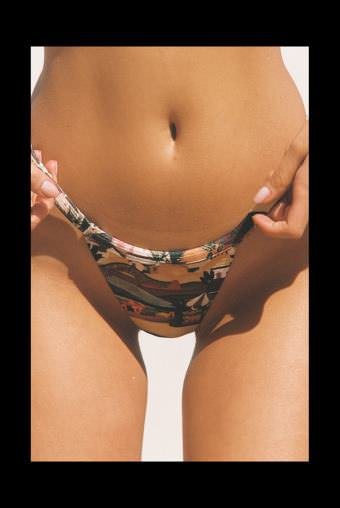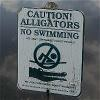65

0   1,906   1 week
NOW-HERE-NO-WHERE53

0   1,914   1 week
NOW-HERE-NO-WHERE39

0   1,781   1 week
NOW-HERE-NO-WHERE52

0   1,788   1 week
NOW-HERE-NO-WHERE90

0   5,322   3 weeks
NOW-HERE-NO-WHERE87

0   3,183   6 weeks
NOW-HERE-NO-WHERE82

0   3,002   6 weeks
NOW-HERE-NO-WHERE62

0   2,433   6 weeks
NOW-HERE-NO-WHERE66

0   2,500   6 weeks
NOW-HERE-NO-WHERE70

0   2,503   3 months
Rick71

0   3,583   4 months
NOW-HERE-NO-WHERE59

0   2,841   4 months
NOW-HERE-NO-WHERE75

0   4,837   4 months
NOW-HERE-NO-WHERE83

0   4,921   4 months
NOW-HERE-NO-WHERE115

0   3,630   4 months
NOW-HERE-NO-WHERE99

0   3,312   4 months
NOW-HERE-NO-WHERE93

0   3,258   4 months
NOW-HERE-NO-WHERE94

0   3,033   4 months
NOW-HERE-NO-WHERE98

0   4,232   8 months
NOW-HERE-NO-WHERE87

0   3,849   8 months
Rick-->